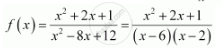CBSE (Arts) Class 11CBSE
Share

# Find the Domain of the Function F(X) = (X^2 + 2x + 1)/(X^2 - 8x + 12) - CBSE (Arts) Class 11 - Mathematics

#### Question

Find the domain of the function  f(x) = (x^2 + 2x + 1)/(x^2 - 8x + 12)

#### Solution

The given function is (x^2 + 2x + 1)/(x^2 - 8x + 12)It can be seen that function f is defined for all real numbers except at x = 6 and x = 2.

Hence, the domain of f is R – {2, 6}.

Is there an error in this question or solution?

#### APPEARS IN

NCERT Solution for Mathematics Textbook for Class 11 (2018 to Current)
Chapter 2: Relations and Functions
Ex. 2.40 | Q: 3 | Page no. 46

#### Video TutorialsVIEW ALL 

Solution Find the Domain of the Function F(X) = (X^2 + 2x + 1)/(X^2 - 8x + 12) Concept: Functions.
S